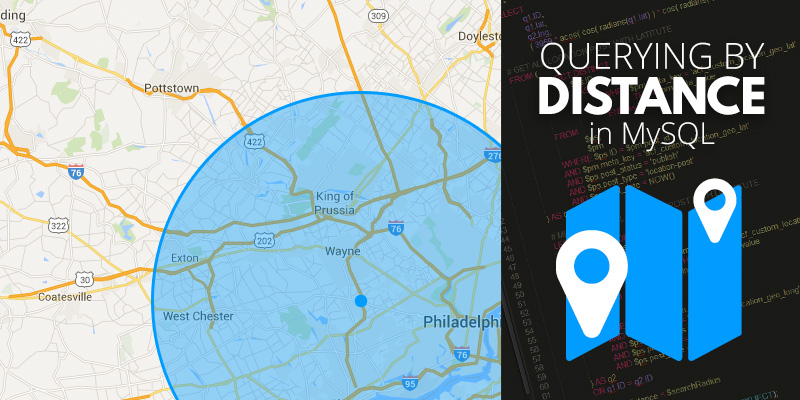# Querying by Distance in MySQL3 min read

Is your website doing everything possible to help your clients find you and get to you? One valuable tool that you can use to accomplish this important goal is to include a “Locations” page on your site. Suppose that your business is a chain of restaurants. In that case, a Locations page can tell customers which of your eateries is closest to the hungry person’s present location.  If you don’t yet have such a page, it’s definitely something worth considering, and here’s how programmers go about building it.

Typically, such a page consists of a search field that allows their users to provide a location, and then returns a list of nearby destinations sorted by distance, often accompanied by a Google Map.

Your only asset is a database table containing all possible locations with columns specifying latitude and longitude. When the user submits their location, you’ll likely utilize the Google Maps API to retrieve the coordinates from an address, which you can then use to compare against your database records.

Conceptually, it’s simple. Query your table and do a little bit of math, and you’ll have your result set. However, if you’re like me, maybe spherical trigonometry isn’t in your area of expertise, and you need to turn to someone else for a drop-in solution. Well, here you go!

``````\$lat = 40.3337075; // user-submitted latitude
\$lon = -75.6374083; // user-submitted longitude
\$dist = 200; // search radius in miles

\$query = "
SELECT loc.*,
3956 * 2 * ASIN(SQRT(POWER(SIN((\$lat-ABS(latitude)) * PI()/180/2),2) + COS(\$lat * PI()/180) * COS(ABS(latitude) * PI()/180) * POWER(SIN((\$lon-longitude) * PI()/180/2),2))) AS distance
FROM locations_table AS loc
WHERE longitude BETWEEN (\$lon-\$dist / ABS(COS(RADIANS(\$lat))*69)) AND (\$lon+\$dist / ABS(COS(RADIANS(\$lat)) * 69))
AND latitude BETWEEN (\$lat-(\$dist/69)) AND (\$lat+(\$dist/69))
GROUP BY loc.id
HAVING distance < \$dist
ORDER BY distance ASC
LIMIT 5
";
``````

Let’s look at this query a little more closely – but not so closely that our heads explode. This example assumes that you have a database table named “locations_table”, and columns named “latitude”, “longitude”, and “id.”

Select all of the columns from table “loc.”

``SELECT loc.*,``

In addition to the locations table, let’s select a custom value called “distance”, which is the calculated distance between the user-submitted location and the location of the current record. The following mathematical sorcery is the Flat Earth Society’s worst nightmare.

``3956 * 2 * ASIN(SQRT(POWER(SIN((\$lat-ABS(latitude)) * PI()/180/2),2) + COS(\$lat * PI()/180) * COS(ABS(latitude) * PI()/180) * POWER(SIN((\$lon-longitude) * PI()/180/2),2))) AS distance``

Query data from the locations table, and give it the alias “loc”.

``FROM locations_table AS loc``

Return only those records where the values of longitude are within a certain range, performing more wizardry that considers the curvature of the earth.

``WHERE longitude BETWEEN (\$lon-\$dist / ABS(COS(RADIANS(\$lat))*69)) AND (\$lon+\$dist / ABS(COS(RADIANS(\$lat)) * 69))``

Also return only those records where the values of latitude are within a certain range.

``AND latitude BETWEEN (\$lat-(\$dist/69)) AND (\$lat+(\$dist/69))``

Consider only those records with a distance value that is lower than the one you specified. This is very important to keep your query as light as possible.

``GROUP BY loc.id HAVING distance < \$dist ORDER BY distance ASC``

Finally, to maintain some semblance of efficiency, limit your result set.

``LIMIT 5``

It is important to note that this is, by nature, a very heavy and inefficient query. It becomes exponentially slower as you increase the value of \$dist, so I’d keep a nice sensible cap on both that value and LIMIT to keep things speedy.

If your database table is burgeoning with locations and you run a reasonably high-traffic web site, you’re going to want to strongly consider implementing a caching solution that helps mitigate the load.

We hope you find this bit of logic useful!